Processing ......FreeComputerBooks.com Links to Free Computer, Mathematics, Technical Books all over the World

Design of Heuristic Algorithms for Hard Optimization: With Python Codes
🌠 Top Free C++ Books - 100% Free or Open Source!
• Title Design of Heuristic Algorithms for Hard Optimization: With Python Codes for the Travelling Salesman Problem
• Author(s) Eric D. Taillard
• Publisher: Springer (October 30, 2022); eBook (Creative Commons Licensed)
• Hardcover: 302 pages
• eBook: PDF and ePub
• Language: English
• ISBN-10: 3031137132
• ISBN-13: 978-3031137136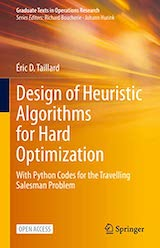Book Description

This open access book demonstrates all the steps required to design heuristic algorithms for difficult optimization. The classic problem of the travelling salesman is used as a common thread to illustrate all the techniques discussed. This problem is ideal for introducing readers to the subject because it is very intuitive and its solutions can be graphically represented. The book features a wealth of illustrations that allow the concepts to be understood at a glance.

• Éric D. Taillard is a professor at the University of Applied Sciences and Arts of Western Switzerland, HEIG-VD campus in Yverdon-les-Bains.
Reviews, Ratings, and Recommendations: Related Book Categories: Read and Download Links:Similar Books:
•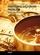Traveling Salesman Problem, Theory and Applications

This book is a collection of current research in the application of evolutionary algorithms and other optimal algorithms to solving the Travelling Salesman Problem (TSP). Most importantly, it presents both theoretical as well as practical applications of TSP,

•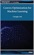Convex Optimization for Machine Learning (Changho Suh)

This book covers an introduction to convex optimization, one of the powerful and tractable optimization problems that can be efficiently solved on a computer. The goal is to help develop a sense of what convex optimization is, and how it can be used.

•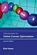Introduction to Online Convex Optimization (Elad Hazan)

This book presents a robust machine learning approach that contains elements of mathematical optimization, game theory, and learning theory: an optimization method that learns from experience as more aspects of the problem are observed.

•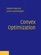Convex Optimization (Stephen Boyd, et al.)

On recognizing convex optimization problems and then finding the most appropriate technique for solving them. It contains many worked examples in fields such as engineering, computer science, mathematics, statistics, finance, and economics.

•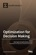Optimization for Decision Making: Linear and Quadratic Models

This book illustrates how to formulate real world problems using linear and quadratic models; It focus on developing modeling skills to support valid decision making for complex real world problems.

•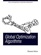Global Optimization Algorithms - Theory and Application

This book is devoted to global optimization algorithms, which are methods to find optimal solutions for given problems. It especially focuses on Evolutionary Computation by discussing evolutionary algorithms, genetic algorithms, Genetic Programming, etc.

•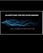Algorithms for Decision Making (Mykel Kochenderfer, et al)

This textbook provides a broad introduction to algorithms for decision making under uncertainty, covering the underlying mathematical problem formulations and the algorithms for solving them.

•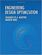Engineering Design Optimization (Joaquim R. Martins, et al)

The philosophy of this book is to provide a detailed enough explanation and analysis of optimization methods so that readers can implement a basic working version. Practical tips are included for common issues encountered in practical engineering design optimization.

•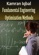Fundamental Engineering Optimization Methods (Kamran Iqbal)

This bookcovers the fundamentals of commonly used optimization methods in engineering design. These include graphical optimization, linear and nonlinear programming, numerical optimization, and discrete optimization.

•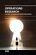Operations Research - the Art of Making Good Decisions

This book is dedicated to operations research of broad applications, it provides a tool for efficient use of natural resources. Both theory and practice of operations research and its related concepts are covered in the book.

•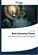Basic Queueing Theory: System Performance Modeling

Queueing Theory is one of the most commonly used mathematical tool for the performance evaluation of systems. The aim of the book is to present the basic methods, approaches in a Markovian level for the analysis of not too complicated systems.

•Knapsack Problems: Algorithms and Computer Implementations

The text fully develops an algorithmic approach to Knapsack Problems without losing mathematical rigor. It provides a comprehensive overview of the methods for solving Knapsack Problems (KP), its variants and generalizations.

Book Categories
 :All CategoriesTop Free BooksRecent BooksMiscellaneous BooksComputer EngineeringComputer LanguagesComputer ScienceData Science/DatabasesJava and Java EE (J2EE)Linux and UnixMathematicsMicrosoft and .NETMobile ComputingNetworking and CommunicationsSoftware EngineeringSpecial TopicsWeb Programming
Other Categories